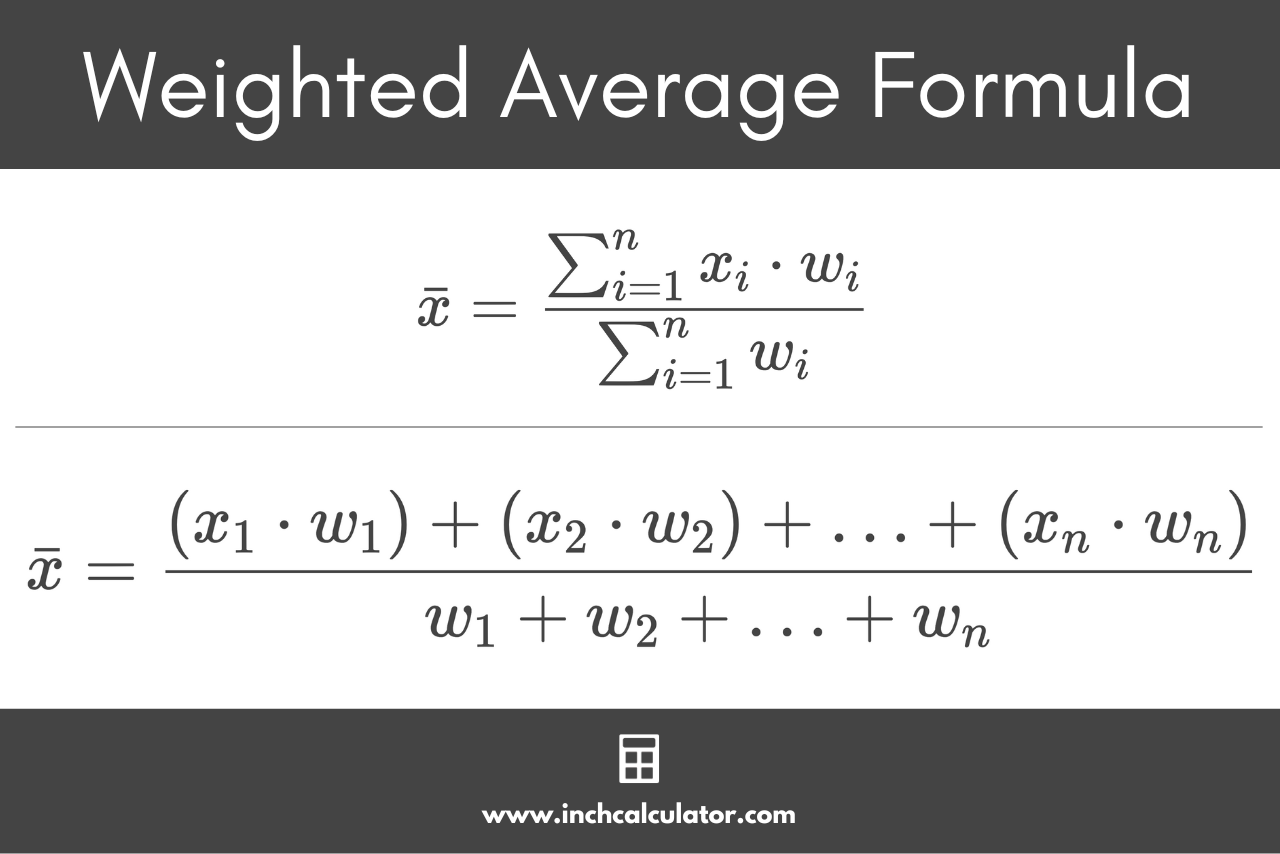# Weighted Average Calculator

Enter the values in a data set and their respective weights to calculate the weighted average.

Value Weight x1 w1 x2 w2 x3 w3 x4 w4 x5 w5 x6 w6 x7 w7 x8 w8 x9 w9 x10 w10 x11 w11 x12 w12 x13 w13 x14 w14 x15 w15 x16 w16 x17 w17 x18 w18 x19 w19 x20 w20 x21 w21 x22 w22 x23 w23 x24 w24 x25 w25

## Weighted Average:

### Steps to Find the Weighted Average

To find the weighted mean, use the following formula:

\bar{x} = \frac{\left (x_{1} \cdot w_{1} \right ) + \left (x_{2} \cdot w_{2} \right ) + ... + \left (x_{n} \cdot w_{n} \right )}{w_{1} + w_{2}+ ... + w_{n}}
[formula may scroll beyond screen]
Learn how we calculated this below

## How to Find a Weighted Average

A weighted average, sometimes called a weighted mean, is an average value that reflects the varying amount of importance of each value in a data set. This is different from the arithmetic mean, where each value contributes equally when calculating the average.

Weighted averages are often used for applications such as sports statistics, class grades and GPA, job performance, credit scores, averaging the cost of capital (e.g. WACC), or calculating stock cost basis.

A classic example of weighted averages is the school course, where homework is worth a smaller portion of the grade than the final exam.

### Weighted Average Formula

To calculate a weighted average or weighted mean, you can use the following formula:

\bar{x} = \frac{\sum_{i=1}^{n}x_{i} \cdot w_{i}}{\sum_{i=1}^{n}w_{i}}

This weighted average formula can be expressed more simply:

\bar{x} = \frac{\left (x_{1} \cdot w_{1} \right ) + \left (x_{2} \cdot w_{2} \right ) + … + \left (x_{n} \cdot w_{n} \right )}{w_{1} + w_{2}+ … + w_{n}}
[formula may scroll beyond screen]

Thus, the weighted average is equal to the sum of the products of each weight and its value, divided by the sum of the weights.For example, let’s use the weighted average formula to calculate a student’s grade average with the following courses and grades:

• class 1: 4 credits B (3.0)
• class 2: 3 credits A (4.0)
• class 3: 4 credits A- (3.7)
• class 4: 3 credits B+ (3.3)
GPA = \frac{\left (3.0 \cdot 4 \right ) + \left (4.0 \cdot 3 \right ) + \left (3.7 \cdot 4 \right ) + \left (3.3 \cdot 3 \right )}{4+3+4+3}
[formula may scroll beyond screen]
GPA = \frac{12.0+12.0+14.8+9.9}{4+3+4+3}
[formula may scroll beyond screen]
GPA = \frac{48.7}{14}
GPA = 3.48

This student’s GPA is 3.48.

This example should demystify how weighted averages work. You might also be interested in learning more about the geometric mean.